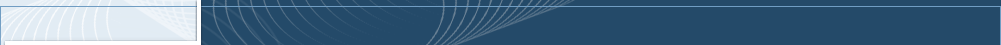About Me Publications Graduate Students Courses Software & Hardware Xampling SAMPL Lab Press Watch Events Links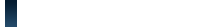Home Contact Me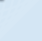Xampling Pulse Streams, Medical Imaging Innovation Rate Sampling of Pulse Streams with Application to Ultrasound Imaging Ronen Tur, Noam Wagner, Yonina C. Eldar & Zvi Friedman Introduction Signals comprised of a stream of short pulses appear in many applications including bio-imaging and radar. The recent finite rate of innovation framework, has paved the way to low rate sampling of such pulses by noticing that only a small number of parameters per unit time are needed to fully describe these signals. Unfortunately, for high rates of innovation, existing sampling schemes are numerically unstable. In this work we propose and demonstrate a novel sampling approach which leads to stable recovery even in the presence of numerous pulses. We derive a condition on the sampling kernel which allows perfect reconstruction from the minimal number of samples. We then propose a compactly supported class of filters, satisfying this condition. Our solution is noise robust and numerically stable even for a large number of pulses. Finally, we apply our techniques on ultrasound imaging data, and show that substantial rate reduction with respect to traditional ultrasound sampling schemes can be achieved. Signal Model Consider a tau-periodic stream of pulses, defined aswhere h(t) and tau are the known pulse shape and period, respectively, and {tl,al} are the unknown delays and amplitudes. The goal is to sample and reconstruct x(t) efficiently. This is done by expanding the signal to its Fourier series, and noticing that the Fourier series coefficients are of the form: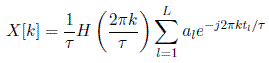The right hand side of this equation is a sum of exponentials with frequencies {tl}, which can be found using standard spectral analysis methods as long as we have a set of Fourier coefficients {X[k]}, with cardinality greater than 2L. The challenge is to obtain the set of Fourier coefficients from time domain samples of the signal x(t). X-ADC: SoS Filters In order to obtain the set {X[k]}, we propose uniformly sampling the signal with a any sampling kernel satisfying: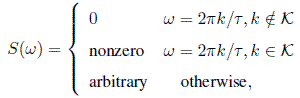where К denotes the index set of the Fourier Coefficients. For this filter choice, it can be shown that the Fourier coefficients can be obtained from the samples by simple matrix inversion, as long as the number of samples is larger than the cardinality of the index set К. Following the general condition presented above, we propose a compactly supported filter which consists of a sum of sinc functions in the frequency domain:where bk are free parameters of the filter. Switching to the time domain it is evident that the filter is compactly supported: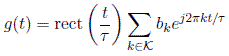The SoS filter results in a system which is highly robust to noise. In addition, the compact support of the SoS filter class enables extension of our solution to the infinite setting. Infinite and Finite Pulse Streams Consider now the case of an infinite stream of pulses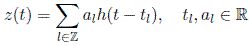We assume that the infinite signal has a bursty character, i.e., the signal has two distinct phases: a) bursts of maximal duration tau containing at most L pulses, and b) quiet phases between bursts. For the sake of clarity we begin with the case  h(t)=δ(t).Consider uniformly sampling z(t) with a filter comprising three periods of g3P(t) = g(t-tau) + g(t) + g(t+tau). If the minimal spacing between any two consecutive bursts is 3T/2, then we are guaranteed that each sample taken during the burst is influenced by one burst only. Here we exploited the compact support of the SoS filter. Once we reduced the infinite problem to a sequence of finite pulse streams, it can be shown that the samples obtained by the g3P(t) sampling kernel form a set of equations which allows to obtain the set of Fourier coefficients exactly as in the periodic case, for each burst independently. Therefore the unknown delays and amplitudes can be determined throughout the infinite signal z(t). The extension to arbitrary h(t) is possible as long as the pulse-shape has finite support, which is a rather weak condition, since our primary interest is in very short pulses which have wide, or even infinite, frequency support. The derivation in this section obviously holds for finite pulse streams, as a simple special case. Note that in the infinite case the SoS approach is not a minimal rate sampling scheme. In order to achieve the minimal possible rate, the approach in Multichannel pulse stream acquisition may be chosen, in the expense of implementation difficulties related to multichannel schemes. Ultrasound Imaging Application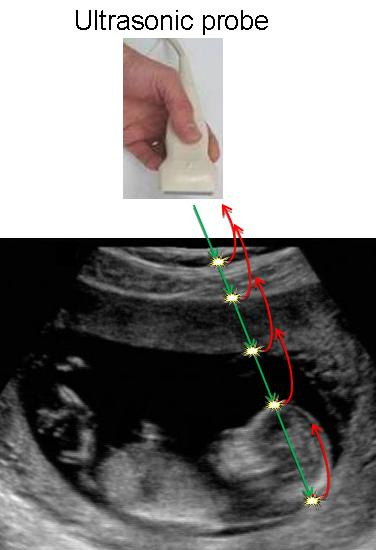An interesting application of our scheme is ultrasound imaging, in which the signal received from the tissue under test comprises a stream of short Gaussian pulses. Applying our scheme on data recorded with GE Healthcare's Vivid-i system, we reconstructed the original signal as depicted in the figure below. The reconstruction is based on 17 samples only, whereas current ultrasonic imaging systems use for the same scenario 4000 samples, emphasizing the potential of our scheme in reducing sampling rate in such systems.Presentation Reference N. Wagner, Y. C. Eldar, A. Feuer, and Z. Friedman, "Compressed Beamforming in Ultrasound Imaging", IEEE Transactions on Signal Processing, vol. 60, issue 9, pp.4643-4657, Sept. 2012. R. Tur, Y. C. Eldar & Z. Friedman, "Innovation Rate Sampling of Pulse Streams with Application to Ultrasound Imaging", IEEE Transactions on Signal Processing, vol. 59, no. 4, pp. 1827-1842, Apr. 2011. Software Download Download a Matlab package demonstrating the proposed method for a stream of Gaussian pulsesDescription: This simulation demonstrates the proposed sampling and reconstruction approach, for a stream of Gaussian pulses. In addition, our method is applied to real ultrasound imaging data, where the pulse is modeled as a Gaussian. Since ultrasonic data is quite noisy, we oversample (typically a factor of 2), apply hard-thresholding to the received signal, and use Cadzow's denoising method. Analog filtering is replaced by digital processing via a dense grid mimicking the corresponding analog operations. Installation: Unzip all files to a directory of your choice. Usage: Follow the instructions in "SoS_GaussianPulse_Description.doc". Download a Matlab package for performance analysis of the proposed method in the presence of noise Description: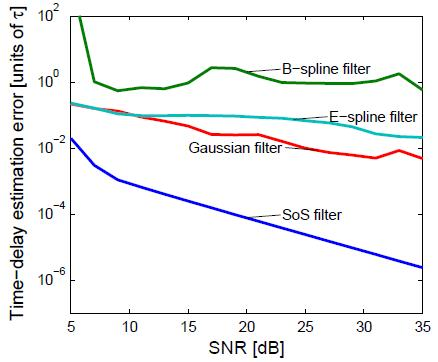This simulation analyzes the performance of our method in the presence of noise, compared to previous approaches. The signal is chosen to be a stream of Dirac delta functions, which allows to calculate the resulting samples analytically. At this stage noise is added to the samples to give the desired SNR. The MSE of time-delay estimation is then given for each method as a function of SNR. Installation: Unzip all files to a directory of your choice. Usage: Follow the instructions in "SoS_Noise_Performance.doc". XamplingThe Big PictureWideband Communication and Cognitive RadioMultipath Channel IdentificationPulse Streams with Medical ApplicationSub-Nyquist Ultrasound ImagingMultichannel Pulse StreamsSuper-Resolution RadarCopyright © 2010 Professor Yonina Eldar. All Rights Reserved.